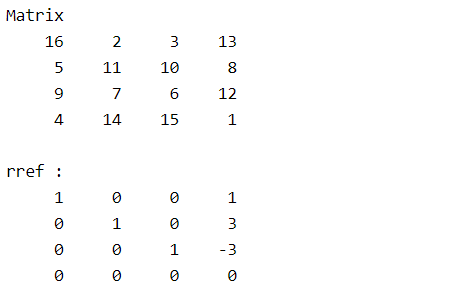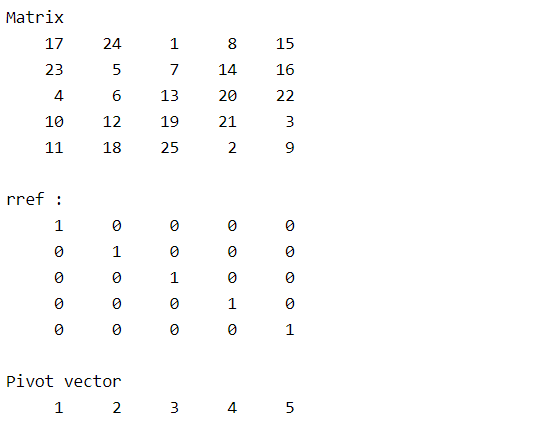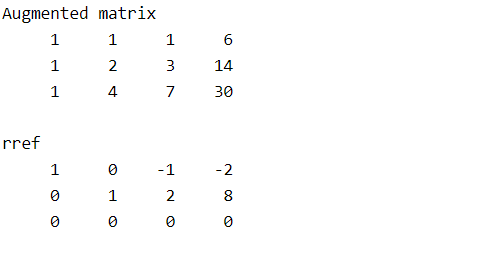Related Articles

# Reduced Row Echelon Form (rref) Matrix in MATLAB

• Last Updated : 14 May, 2021

Reduced Row Echelon Form of a matrix is used to find the rank of a matrix and further allows to solve a system of linear equations. A matrix is in Row Echelon form if

• All rows consisting of only zeroes are at the bottom.
• The first nonzero element of a nonzero row is always strictly to the right of the first nonzero element of the row above it.

Example :A matrix can have several row echelon forms. A matrix is in Reduced Row Echelon Form if

• It is in row echelon form.
• The first nonzero element in each nonzero row is a 1.
• Each column containing a nonzero as 1 has zeros in all its other entries.

Example:Where a1,a2,b1,b2,b3 are nonzero elements.

A matrix has a unique Reduced row echelon form. Matlab allows users to find Reduced Row Echelon Form using rref() method. Different syntax of rref() are:

• R = rref(A)
• [R,p] = rref(A)

Let us discuss the above syntaxes in detail:

### rref(A)

It returns the Reduced Row Echelon Form of the matrix A using the Gauss-Jordan method.

## Matlab

 % creating a matrix using magic(n)% generates n*n matrix with values% from 1 to n^2 where every row sum% is equal to every column sumA = magic(4);disp("Matrix");disp(A);  % Reduced Row Echelon Form of ARA = rref(A);disp("rref :");disp(RA);

Output :### rref(A)

• It returns Reduced Row Echelon Form R and a vector of pivots p
• p is a vector of row numbers that has a nonzero element in its Reduced Row Echelon Form.
• The rank of matrix A is length(p).
• R(1:length(p),1:length(p)) (First length(p) rows and length(p) columns in R) is an identity matrix.

## Matlab

 % creating a matrix using magic(n)% generates n*n matrix with values % from 1 to n^2 where every row sum% is equal to every column sumA = magic(5);disp("Matrix");disp(A);  % Reduced Row Echelon Form of A[RA,p] = rref(A);disp("rref :");disp(RA);  % Displaying pivot vector pdisp("Pivot vector");disp(p);

Output :### Finding solutions to a system of linear equations using Reduced Row Echelon Form:

The System of linear equations isCoefficient matrix A isConstant matrix B isThen Augmented matrix [AB] is## Matlab

 % Coefficient matrixA = [1  1  1;     1  2  3;     1  4  7];       % Constant matrixb = [6 ;14; 30];  % Augmented matrixM = [A b];disp("Augmented matrix");disp(M)  % Reduced Row echelon form of % Augmented matrixR = rref(M);disp("rref");disp(R)

Output :Then the reduced equations areIt has infinite solutions, one can be.

My Personal Notes arrow_drop_up